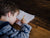# What Do Children Learn in Grade 3 Maths?Children start learning mathematical and numerical concepts at an early age, from reading numbers to understanding addition and subtraction. All the way from kindergarten to high school, maths is an important part of a child’s development and literacy.

By supporting their foundational knowledge, children will be prepared for maths problems that they may come across in daily life. This includes things like general problem solving and money management.

In Grade 3 curriculums across the country, children will be introduced to new mathematical concepts and problems, such as measurement, geometry, and probability. This is an important time for expanding their knowledge and building confidence, which will help to set them up for future success.

Children around the age of 8 and 9 benefit from many different experiences in drawing, counting, and talking about numbers. From a range of learning activities, to opportunities to practise and test their knowledge, learning through comprehensive maths worksheets and workbooks is a great way for children to learn.

These types of Year 3 maths worksheets are a big part of the maths curriculum in Australian schools. Below are the main areas of learning.

## What children learn in Grade 3 Maths:

### Numbers and algebra

In primary school, children are introduced to formal written algorithms and number problems after they have gained a good understanding of basic concepts, such as placing value. Things like patterns and algebra are incorporated into their learning to help them develop algebraic thinking.

Children are taught things such as important new maths words and written numbers, fractions and decimals, and understanding values in different formulae. In Year 3 maths worksheets, this appears as bar graphs and corresponding counting activities, visual representation of simple fractions, and line graphs to understand money and value.

### Measurement and geometry

Measurement and geometry help children to understand shapes and their properties, geometric reasoning, and units of measurement like length, mass, weeks, and months. These types of concepts are essential for understanding the world around them, and this stage of their learning is particularly important.

Children are taught things like the features of angles, triangles, rectangles, and circles, how to identify different measurement words and concepts, and how to read directions and understand how they work.

### Statistics and probability

Learning to read data and understand how this affects chance and probability is a key learning in Grade 3 mathematics. These concepts are introduced simply, such as presenting several ways to display data graphically (such as rows and columns, bar graphs, and line graphs), and activities for reading and interpreting this data.

In the primary curriculum, children build their curiosity about probability and statistics, with the aim to confidently interpret and understand data outputs and be skilled in using data in their work and learning.

## How Fitzroy Maths workbooks can help:

We have developed a series of primary school maths books to thoroughly cover the Australian Curriculum for Year 3. The newly revised Fitzroy Maths workbooks 16-20 introduces essential mathematical and numerical concepts and maths words throughout. This is to enable students to respond to maths problems in tests and future maths books. From times tables to verbal and graphic maths problems, these books are the perfect accompaniment for a child's development, both in the classroom and at home.

Get a sneak peek inside these specially designed books here.

All of our Fitzroy Maths workbooks are specifically designed to help students in their development, from beginner up to middle primary level. The workbooks use the same step-by-step principle and logical sequencing as our Fitzroy Readers

Learn all about our other Fitzroy Maths workbooks preceding and following the Year 3 maths worksheets here.